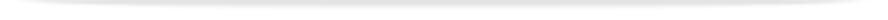units

ENG2092

Faculty of Engineeringprint version

This unit entry is for students who completed this unit in 2016 only. For students planning to study the unit, please refer to the unit indexes in the the current edition of the Handbook. If you have any queries contact the managing faculty for your course or area of study.

ENG2092 - Advanced engineering mathematics B

6 points, SCA Band 2, 0.125 EFTSL

Refer to the specific census and withdrawal dates for the semester(s) in which this unit is offered.

Faculty

Engineering

Coordinator(s)

Dr John Head (Clayton); Mr Nader Kamrani(Malaysia)

Offered

Clayton

• Second semester 2016 (Day)

Malaysia

• Second semester 2016 (Day)

Synopsis

Complex analysis: introduction to functions of complex variables and the manipulation, differentiation and integration of complex functions, line integrals in the complex plane. Integral transforms: introduction of Laplace transforms and their application to ordinary differential equations. Statistics: probability density function and distribution function of random variables, joint density function of multivariate random functions, expectation and confidence limits of random variables.

Outcomes

On completing this unit, students will be able to manipulate elementary functions of complex variables (eg multiplication, division, root finding); manipulate exponential and trigonometric functions of complex variables; calculate derivatives and integrals of elementary functions of complex variables; calculate line integrals on the complex plane, apply Cauchy's integral theorem; employ simple Laplace transforms to solve ordinary differential equations; appreciate the representation of random variables through the distribution and density functions; calculate the expected value of a random variable; find the joint distribution of a multivariate random function; develop inference and confidence limits of random variables; calculate linear regression and correlations.

Assessment

Assignments and test: 30%
Examination (3 hours): 70%

3 hours lectures, 2 hours practice classes and 7 hours of private study per week